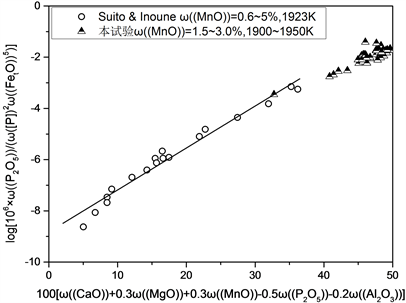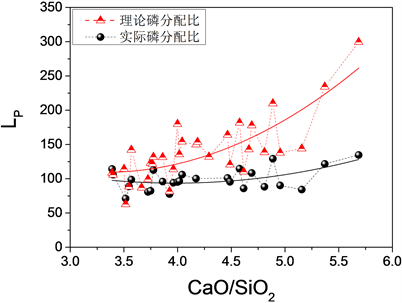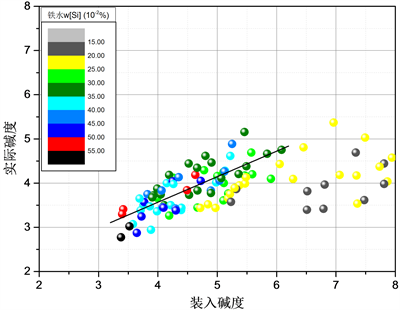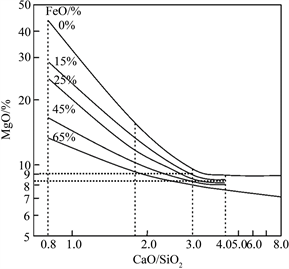﻿ 350 t转炉终点炉渣成分控制研究

# 350 t转炉终点炉渣成分控制研究Study on Final Slag Composition Control of 350 t Converter

Abstract: An investigation was carried out on control of phosphorus content in 350 t Combined Blowing Con-verter of Zhanjiang Iron & Steel. The reason for low phosphorus distribution ratio between slag and steel at the end of steelmaking was analyzed thermodynamically. In the meantime, the suggestions for adjusting composition of converter slag were given, and the optimum basicity of slag to get the corresponding dephosphorization rate and phosphorus distribution ratio was determined. The results showed that, under the condition of existing technology, high basicity and the great amount of slag resulted in relatively large difference between the measured and theoretical phosphorus distribution ratio. The calculation indicated that, for the existing process, the amount of slag to be added into converter should be controlled in 70 kg/t.

1. 引言

2. 试验条件

3. 结果分析与讨论

3.1. 转炉生产取样分析

Table 1. Variation of phosphorus content in molten steel before and after converter steelmakingTable 2. Composition of slag at the end of converter steelmaking (%)

3.2. 转炉脱磷的热力学分析

$\frac{1}{2}{\text{P}}_{2}\left(\text{g}\right)+\frac{5}{4}{\text{O}}_{2}\left(\text{g}\right)+\frac{3}{2}\left({\text{O}}^{2-}\right)=\left({\text{PO}}_{4}^{3-}\right)$ (1)

${C}_{{\text{PO}}_{4}^{3-}}=\frac{{K}_{{\text{PO}}_{4}^{3-}}{\left({a}_{{\text{O}}^{2-}}\right)}^{3/2}}{{f}_{{\text{PO}}_{4}^{3-}}}=\frac{\omega \left(\left({\text{PO}}_{4}^{3-}\right)\right)}{{P}_{{\text{P}}_{2}}^{1/2}\cdot {P}_{{\text{O}}_{2}}^{5/4}}$ (2)

$\frac{1}{2}{\text{P}}_{2}\left(\text{g}\right)=\left[\text{P}\right]\text{\hspace{0.17em}}\text{\hspace{0.17em}}\text{\hspace{0.17em}}\text{\hspace{0.17em}}\Delta {G}^{\theta }=-157700+5.4T$ (3)

$\frac{1}{2}{\text{O}}_{2}\left(\text{g}\right)=\left[\text{O}\right]\text{\hspace{0.17em}}\text{\hspace{0.17em}}\text{\hspace{0.17em}}\text{\hspace{0.17em}}\Delta {G}^{\theta }=-117110-3.39T$ (4)

$\mathrm{lg}{C}_{{\text{PO}}_{4}^{3-}}=\mathrm{lg}{L}_{\text{P}}-\mathrm{lg}{f}_{\text{P}}-2.5\mathrm{lg}{a}_{\text{O}}+\mathrm{lg}{K}_{\text{P}}+2.5\mathrm{lg}{K}_{\text{O}}+0.49$ (5)

$\begin{array}{l}\mathrm{log}\left[{10}^{6}×\omega \left(\left({\text{P}}_{\text{2}}{\text{O}}_{\text{5}}\right)\right)/\left(\omega {\left(\left[\text{P}\right]\right)}^{2}\cdot \omega {\left(\left({\text{Fe}}_{\text{t}}\text{O}\right)\right)}^{5}\right)\right]\\ =14.5\left[\omega \left(\left(\text{CaO}\right)\right)+0.3\omega \left(\left(\text{MgO}\right)\right)+0.3\omega \left(\left(\text{MnO}\right)\right)-0.5\omega \left(\left({\text{P}}_{\text{2}}{\text{O}}_{\text{5}}\right)\right)\\ \text{\hspace{0.17em}}\text{\hspace{0.17em}}\text{ }+1.2\omega \left(\left(\text{CaF}\right)\right)-0.2\omega \left(\left({\text{Al}}_{\text{2}}{\text{O}}_{\text{3}}\right)\right)\right]+22810/T-20.51\end{array}$ (6)Figure 1. Comparison of results obtained by Suito and in this work

$\begin{array}{c}\mathrm{log}\omega \left(\left(\text{P}\right)\right)/\omega \left(\left[\text{P}\right]\right)=7.2\left[\omega \left(\left(\text{CaO}\right)\right)+0.3\omega \left(\left(\text{MgO}\right)\right)+0.2\omega \left(\left(\text{MnO}\right)\right)\\ \text{\hspace{0.17em}}\text{\hspace{0.17em}}+0.6\omega \left(\left({\text{P}}_{\text{2}}{\text{O}}_{\text{5}}\right)\right)+1.2\omega \left(\left(\text{CaF}\right)\right)-0.5\omega \left(\left({\text{Al}}_{\text{2}}{\text{O}}_{\text{3}}\right)\right)\right]\\ \text{\hspace{0.17em}}\text{\hspace{0.17em}}+11570/T-15.52+2.5\mathrm{log}\omega \left(\left(\text{P}\right)\right)\end{array}$ (7)Figure 2. Comparison of measured and theoretical Lp values at the end of conwerter steelmaking

4. 转炉渣的调节

4.1. 装入碱度的调节

${R}_{装入}=\left(80%{W}_{石灰}+50%{W}_{石灰石}+30%{W}_{轻烧}+20%{W}_{轻烧压球}\right)/\left({W}_{铁水}\cdot \omega \left(\left[\text{Si}\right]\right)\cdot 68/28\right)$ (8)Figure 3. Comparison of measured and theoretical basicity of converter slag

4.2. 炉渣成分的调节Figure 4. Solubility of saturated MgO for slag system CaO-SiO2-FeO-MgO (1600˚C)

4.3. 渣量的调节

${W}_{m}\left(\omega {\left(\left[\text{P}\right]\right)}_{0}-\omega \left(\left[\text{P}\right]\right)\right)={W}_{s}\left(\omega \left(\left(\text{P}\right)\right)-\omega \left({\left(\text{P}\right)}_{0}\right)\right)$ (9)

$\begin{array}{c}{W}_{s}={W}_{m}\left(\omega {\left(\left[\text{P}\right]\right)}_{0}-\omega \left(\left[\text{P}\right]\right)\right)/\left(\omega \left(\left(\text{P}\right)\right)-\omega \left({\left(\text{P}\right)}_{0}\right)\right)\\ =350×\left(0.10%-0.01%\right)÷\left(3.0%×62÷142\right)=24\left( t \right)\end{array}$

5. 结论

1) 转炉终点渣钢磷分配比理论值与实际值的趋势是一致的，但存在一定的差距，且随着炉渣碱度的增大，二者之间的差距不断增大，这说明炉渣的脱磷能力未能充分的利用。

2) 将碱度控制在3.0~4.0之间，既能满足转炉终点炉渣的冶炼性能，又可以将渣中MgO的饱和溶解度控制在较低水平(9%~12%)，还可以减少炉渣成分波动对炉况的影响。

3) 当铁水硅含量在0.25%以上时，将装入碱度控制在3.5~4.5之间，即可保证转炉终点炉渣碱度在3.0~4.0之间，对于低硅铁水为保证渣量可适当提高装入碱度。

4) 目前湛钢平均渣量在30 t左右，比理论计算渣量偏大，这说明在实际生产中还需要进一步改善转炉化渣效果，充分提高转炉渣的磷容量，以减少渣量。

 王能贤. 磷对钢冲击韧性的影响[J]. 特殊钢, 1993(4): 53.

 吴伟, 邹宗树. 转炉冶炼终点炉渣氧化性和碱度的研究[J]. 炼钢, 2004, 20(3): 28-32.

 刘飞, 管挺. 转炉吹炼过程炉渣的变化及其对脱磷的影响[J]. 炼钢, 2013, 29(6): 23-28.

 Wagner, C. (1975) Concept of Basicity of Slags. Metallurgical Transactions B, 6, 405.
https://doi.org/10.1007/BF02913825

 Nakamura, S., Tsukihashi, F. and Sano, N. (1993) Phosphorus Partition between CaOsatd-BaO-SiO2--FetO Slags and Liquid Iron at 1873K. ISIJ International, 33, 53.
https://doi.org/10.2355/isijinternational.33.53

 Suito, H. and Inoue, R. (1995) Thermodynamic Assessment of Hot Metal and Steel Dephosphorization with MnO- Containing BOF Slags. ISIJ International, 35, 258-265.
https://doi.org/10.2355/isijinternational.35.258

 潘贻芳, 杜玲. 转炉炉渣的渣化度[J]. 钢铁, 1994, 29(8): 13-15.

 朱荣, 董履仁, 张鉴. CaO-SiO2-FeO-Fe2O3-MgO渣系MgO饱和溶解度的研究[J]. 炼钢, 1998, 5(11): 24-26.

 周兆保, 李素梅, 程官江. 安钢转炉渣中MgO的饱和溶解度分析[J]. 耐火材料, 2000(4): 248-252.

 代文彬, 李宇, 苍大强, 等. 转炉钢渣热态改性对镁质耐火材料的侵蚀行为[J]. 耐火材料, 2013, 2(8): 12-15.

 Tayed, M.A., Assis, A.N., Sridhar, S., et al. (2015) MgO Solubility in Steelmaking Slags. Metallur-gical and Materials Transaction B, 46, 1112-1114.
https://doi.org/10.1007/s11663-015-0352-8

 Suito, H. and Inoue, R. (2014) Experimental Measurement of the Solubility of MgO in Metallurgical Slags to Control the Slag-Induced Corrosion of Periclase-Carbon Refractories. Refractories & Industrial Ceramics, 55, 114-116.
https://doi.org/10.1007/s11148-014-9671-6

Top